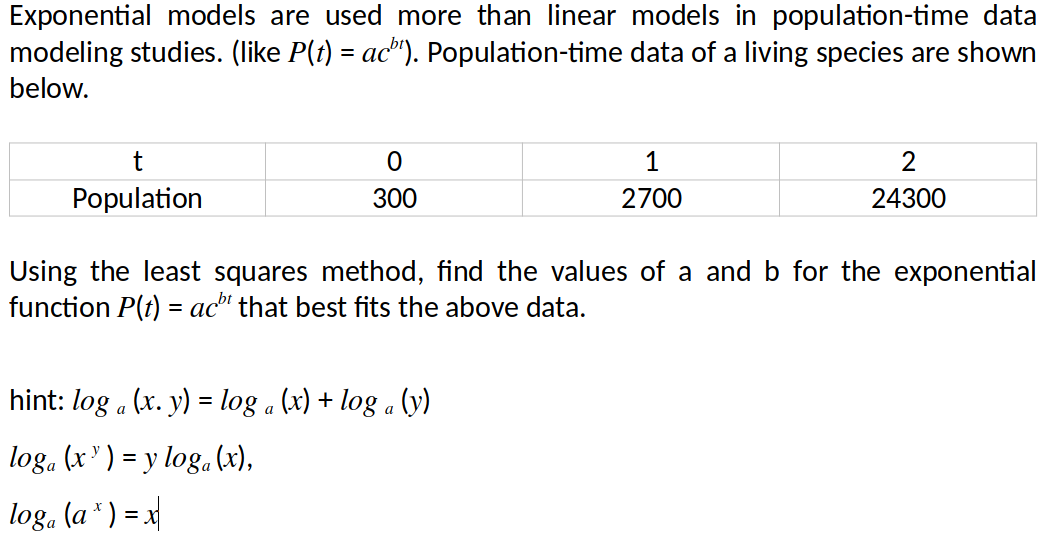Home / Expert Answers / Statistics and Probability / population-time-least-squares-method-exponential-exponential-models-are-used-more-than-linear-pa639

# (Solved): Population-time, least squares method, exponential. Exponential models are used more than linear ...

Population-time, least squares method, exponential.Exponential models are used more than linear models in population-time data modeling studies. (like $$P(t)=a c^{b t}$$ ). Population-time data of a living species are shown below. Using the least squares method, find the values of $$a$$ and $$b$$ for the exponential function $$P(t)=a c^{b t}$$ that best fits the above data. hint: $$\log _{a}(x \cdot y)=\log _{a}(x)+\log _{a}(y)$$ $\begin{array}{l} \log _{a}\left(x^{y}\right)=y \log _{a}(x) \\ \log _{a}\left(a^{x}\right)=x \end{array}$

We have an Answer from Expert